Next: MAXIMUM BALANCED CONNECTED PARTITION Up: Covering and Partitioning Previous: MINIMUM CLIQUE PARTITION   Index

### MINIMUM K-CAPACITATED TREE PARTITION

• INSTANCE: Graph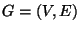, and a weight function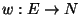.
• SOLUTION: A k-capacitated tree partition of G, i.e., a collection of vertex disjoint subsets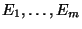of E such that, for each i, the subgraph induced by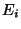is a tree of at least k vertices.
• MEASURE: The weight of the partition, i.e.,.

• Good News: Approximable within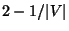.
• Comment: The variation in which the trees must contain exactly k vertices and the triangle inequality is satisfied is approximable within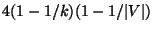. Similar results hold for the corresponding cycle and path partitioning problems with the triangle inequality . Variation in which the trees must contain exactly k vertices and the objective is to minimize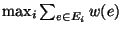is not in APX, but if the triangle inequality is satisfied it is approximable within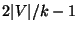. The variation in which the number m of trees is fixed, the trees' sizes are exactly specified, and the triangle inequality is satisfied is approximable within 2p-1 .

Viggo Kann
2000-03-20